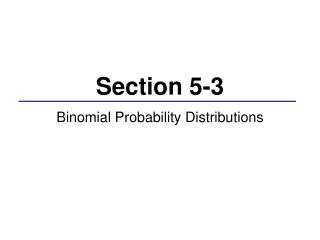DownloadDownload PresentationSection 5-3

# Section 5-3

Télécharger la présentation## Section 5-3

- - - - - - - - - - - - - - - - - - - - - - - - - - - E N D - - - - - - - - - - - - - - - - - - - - - - - - - - -
##### Presentation Transcript

1. Section 5-3 Binomial Probability Distributions

2. What does the prefix “Bi” mean? We will now consider situations where there are exactly two outcomes: • Yes/no to a survey question • Product is defective/not defective • Correct/Wrong response to a multiple choice question • Arrival time for an airline is on time/not on time

3. Conditions for a Binomial Probability Distribution • There are a fixed number of trials, n, that are identical and independent. • Each trial has exactly 2 outcomes: success, S or failure, F • The probability of success must remain the same for each trial.

4. Notation: P(S) = p P(F) = 1 – p = q n = # of trials x = # of successes *success is just what is being measured

5. Example 1 Determine whether the given procedure results in a binomial distribution. • Determining whether each of 3000 heart pacemakers is acceptable or defective. • Surveying people and asking them what they think of the current president. • Spinning the roulette wheel 12 times and finding the number of times that the outcome is an odd number.

6. Methods for finding binomial probabilities • Binomial Probability Formula: • Table A-1, Appendix A • MINITAB

7. Example 2 A report from the Secretary of Health and Human Services stated that 70% of single-vehicle traffic fatalities that occur at night on weekends involve an intoxicated driver. If a sample of 10 single-vehicle traffic fatalities that occur at night on a weekend is selected, find the probability that exactly seven involve a driver that is intoxicated. This is a binomial experiment where: n = 10 x = 7 p = 0.70 q = 0.30

8. Using table generated in MINITAB P(7) = 0.266828

9. How about the probability of at least 8 involving an intoxicated driver? P(at least 8) = P(8) or P(9) or P(10) = 0.233474 + 0.121061 + 0.028248 =0.382783

10. Example 3 In a clinical test of the drug Viagra, it was found that 4% of those in a placebo group experienced headaches. • Assuming that the same 4% rate applies to those taking Viagra, find the probability that among eight Viagra users, three experience headaches.